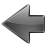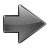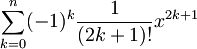jsxgraph.org
p0
JSXGraph
p1
Future trends?
p1a
Future trends?
• Java plugin?
• Flash plugin?
• HTML 5?
p1aa
Future trends?
Which technology to use?
p1b
Future trends?
• Vector graphics
• JavaScript
p2
JavaScript

- All major browsers come with JavaScript

- JavaScript is fast

p3a
JSXGraph
p3
JSXGraph
Open source library for interactive Geometry / Mathematics in the web browser
p5
Features
p4
Features
p6
Free point
p7
Dependent point
B = A + (0,1)
p10
Curves
Space filling Hilbert curve:

Who said JavaScript is slow...?

p11
Turtle graphics
```t = brd.createElement('turtle');
function koch(x,level) {
if (level<1) t.fd(x);
else {
koch(x/3,level-1);
t.lt(60);
koch(x/3,level-1);
t.rt(120);
koch(x/3,level-1);
t.lt(60);
koch(x/3,level-1);
}
}
t.setPos(-250,0);
t.rt(90);
koch(400,7);
```
16385 data points
p27
p32
Geometry
Point
Line
Ray
Segment
Circle
Perpendicular
Intersection
Angle
...
p9
Geometry
Scripting with JavaScript enables recursive constructions
p28
p13
Turtle graphics
Interaction
```t.forward(100);
t.right(90);
t.left(90);
t.back(30);
...
```
p14
Turtle graphics

Lindenmayer systems

Axioms + Rewriting rules + Turtle:
• Space filling curves,
• Plants,
• Penrose tilings,
• ...
p15
Turtle graphics
Simulate differential equations

y0 = s

Δy = α⋅ y⋅ Δt

```dy = alpha.Value()*t.Y()*dt;
t.moveTo([dt+t.X(),dy+t.Y()]);
```
p29
p25
Calculus

Numerical on-the-fly-evaluation of ordinary differential equations (explicit Runge-Kutta).p16
Calculus
Archimedes' approximation of π
p17
Calculus
Power seriesp18
Calculus
• Lagrange interpolation
• Cubic spline interpolation
• Polynomial curve interpolation
p33
Charts
p23
Controlling JSXGraph from the "outside".

There is no "inside" or "outside" of the construction anymore.

p26
Hooks
Not only controlling JSXGraph from the "outside" is possible.

Hooks are functions called by JSXGraph on every update.

With this it is possible to access and display information from "inside" the construction anywhere on the HTML page.

p30
p20
Interactive way

With JSXGraph elements like points can leave a trace.

This is useful to determine geometric loci.

p12
Client/server model

Example for how to extend JSXGraph server side using AJAX:

Determination of geometric loci with CoCoA and matplotlib.

p19
CAS way
p31
p24
Supported file formats
GEONExT:
```JXG.JSXGraph.loadBoardFromFile(
'box',
'cube.gxt',
'Geonext'
);
```
→ GEONExT2
p21
Supported file formats
Intergeo:
```JXG.JSXGraph.loadBoardFromFile(
'box',
'intergeo2.i2g',
'Intergeo'
);
```
JSXGraph has (the first?) JavaScript implementations of gunzip and unzip
p34
Supported file formats
GeoGebra
p35
Supported file formats
GeoGebra:
```JXG.JSXGraph.loadBoardFromFile(
'box',
'feuerbach.ggb',
'Geogebra'
);
```
p22
More examples: http://jsxgraph.org